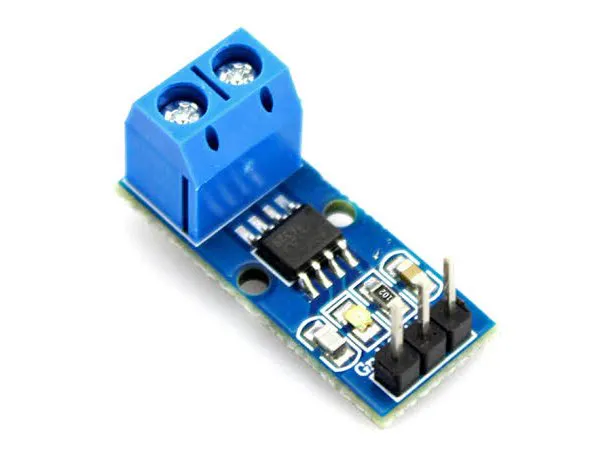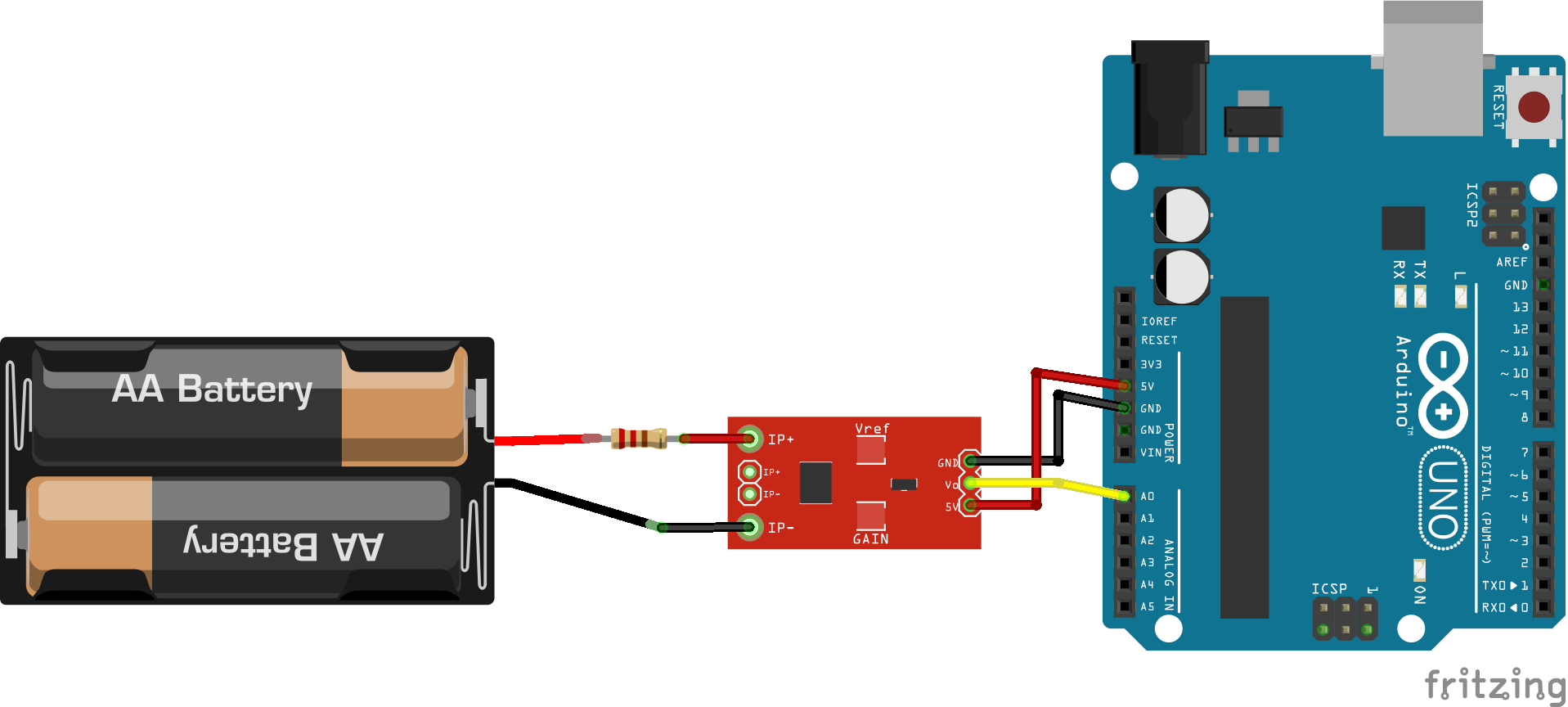# ACS712 Current Sensor © MIT

ACS712 current sensor by Allegro is an Hall effect-based linear current sensor.

• 59,093 views
• 12 respects

## Components and supplies

### Introduction

Hall Effect Sensors are transducer type components that can convert magnetic information into electrical signals for subsequent electronic circuit processing. Generally, current sensors use the Hall Effect to convert current inputs into voltage outputs. In the Hall effect, electrons from an electric current flow through a magnetic field plate. The field then causes the electrons to "push" to one side of the plate and produce a voltage difference between the two sides. The difference in voltage from the side of the plate is the output of the sensor.

ACS712 is a current sensor that can operate on both AC and DC. This sensor operates at 5V and produces an analog voltage output proportional to the measured current. This tool consists of a series of precision Hall sensors with copper lines.

The output of this instrument has a positive slope when the current increases through the copper primary conduction path (from pins 1 and 2 to pins 3 and 4). The internal resistance of the conduction path is 1.2 mΩ.

This sensor has an output voltage of Vcc x 0.5 = 2.5 at the input current 0A and a 5V Vcc power supply. There are three types based on the readable current range, ± 5A, ± 20A, and ± 30A with output sensitivity of each type of 185mV / A, 100mV / A, and 66mV / A respectively.

The output of this current sensor is analog, so to read it, we can directly measure the output voltage using voltmeter or measure it by using a microcontroller like Arduino through Analog Read pin or ADC pin.

### Methodology

For this project, we use a power supply (giving output voltage from 0 to 5 Volt) and a 1 ohm resistor 8 watt to generate current from 0 to 5 Ampere. Later on, we'll measure the voltage output from the ACS712 Current Sensor.

We use Arduino UNO to give ACS712 5V power supply (to 5V pin in ACS712). The ACS712 sensor's ground is also connected to the Arduino UNO ground. For measurement, we connect the + probe of voltmeter to analog output pin in the ACS712.

### Result

We test the sensor by giving 12 measurement points from -2A to 2A input range. The voltage reading of each corresponding measurement is seen as:

Regression of the obtained result shows the relationship

This result shows a 2.5V output at 0A and a 170mV/A slope as expected from the reference sheet.

• Conclusion

In conclusion, the ACS712 current sensor is proven to be able to read electric current and produce an output voltage proportional to the current input. The current-voltage relationship is shown as 2.5V at 0A with a slope around 170mV per Ampere.

## Schematics#### Published on

November 11, 2019

#### Members who respect this project

See similar projects
you might like

#### Measure Any AC Current with ACS712

Project tutorial by SurtrTech

• 48,982 views
• 22 respects

• 15,400 views
• 20 respects

#### The Quantified Cactus: An Easy Plant Soil Moisture Sensor

Project tutorial by Jen Looper

• 16,405 views
• 34 respects

#### Touch Sensor and Sound Sensor Controlling AC/DC Lights

Project showcase by Anbumachi

• 6,146 views
• 8 respects

#### DHT11 Temperature/Humidity Sensor

Project tutorial by Zachary Haslam

• 173,421 views